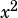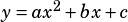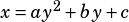Parabola D in the xy-plane has equation x – 2y^2 – 8y – 11 = 0. Which equation shows the x-intercept(s) of the parabola as constants or coefficients?

A) x = 2y^2 + 8y + 11
B) x = 2(y + 2)^2 + 3
C) x – 3 = 2(y + 2)^2
D) y = – √(x – 3)/2 – 2

The answer is A) which makes me think this is really just a “do you know the definition of this term” kind of question (similar to official practice test 4.4.28). Can you explain for those weak in this? TIA!

When a question asks for an intercept or a vertex or some other notable point “as constants or coefficients,” you’ve got a couple options.

The first is to recognize the form that typically gives that information. For example, if you needed to see the x-intercepts of a vertical parabola (the kind we almost always see), you’d look for answer choices in factored form—e.g.,—because that form tells you the x-intercepts. That method works here, but it’s a bit less straightforward than usual because we’re dealing with a horizontal parabola. Basically, the thought process is:

1. Recognize that the equation gives you a horizontal parabola (it’s got aterm instead of anterm)
2. Recognize that the properties of a horizontal parabola should be similar to those of a vertical parabola only the x and y terms will be flipped
3. Know that in a vertical parabola, the standard form——gives you the y-intercept (the c term), so in a horizontal parabola you should be able to get the x-intercept from a similar form
4. The only answer choice in theform is A

The second option is to solve for the number being requested and then look for it in the answer choices. In this case, we want x-intercepts. We can always find a graph’s x-intercepts by setting y equal to zero and solving for x.Now you know you’re looking for an answer choice with an 11 in it. Only choice A has that!